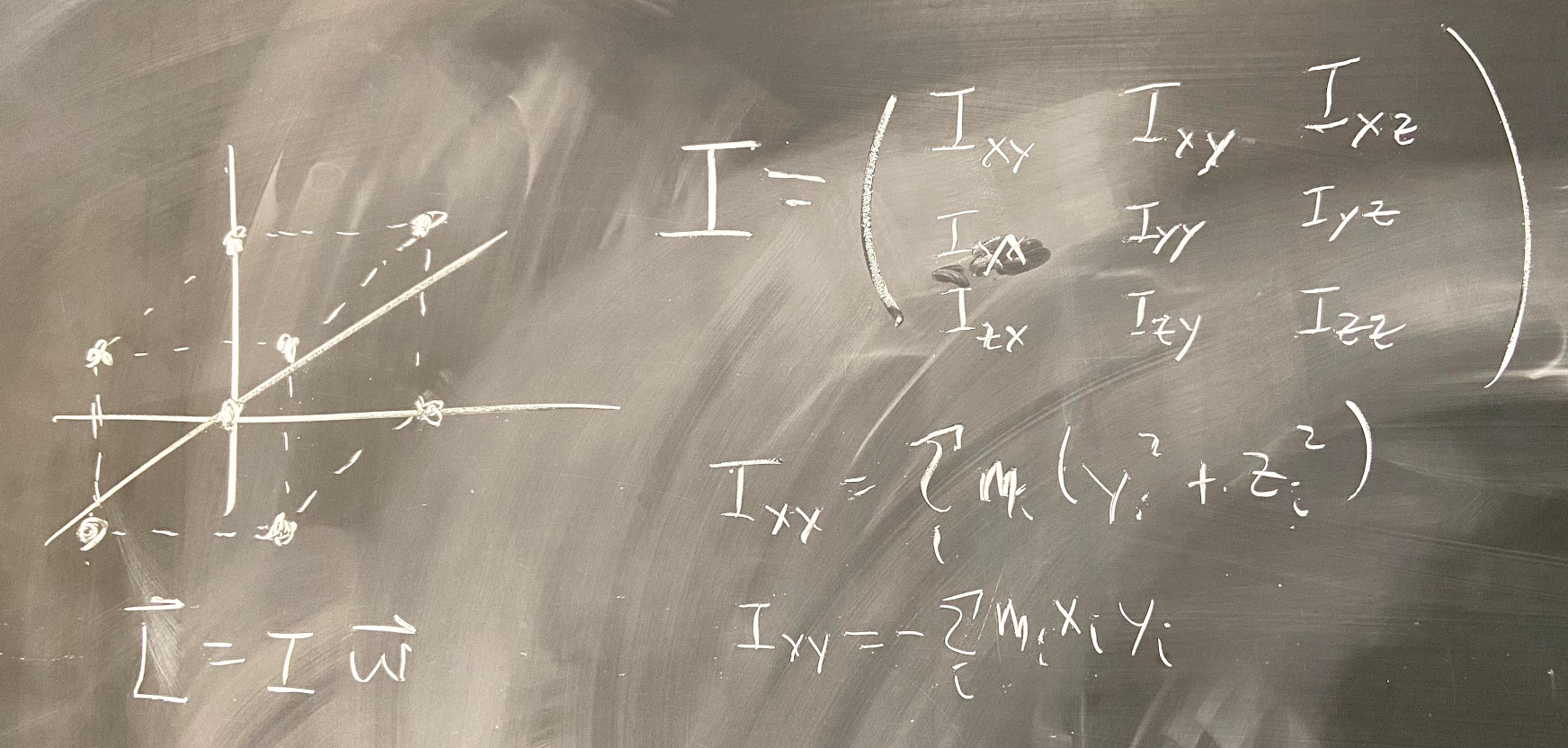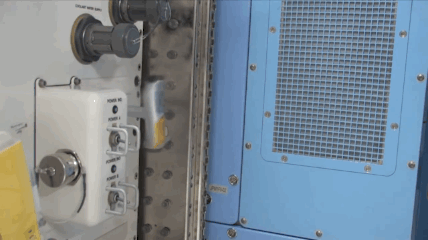# Modeling a Spinning and Flipping Handle Using Springs and PythonPhoto: Rhett Allain

I don’t think that title really explains the problem. It’s called the Dzhanibekov Effect — but that doesn’t really help either. How about this? Imagine you are in the International space station and you unscrew a t-handle really fast. Once the handle leaves the thread, it’s just floating there and spinning. Then this happens (here is the YouTube version):Captured from this Plasma Ben video on YouTube.

Isn’t that crazy? I mean, it’s crazy awesome. It goes against our basic physics intuition. We see this thing spinning and there’s clearly no torque on it — then how does its angular momentum change? The answer is: it doesn’t. Ha.

It all comes down to this definition of angular momentum:

Yes, L is the angular momentum vector and ω is the angular velocity vector. Finally, I is the moment of inertia. We like to think of this equation as being very similar to the linear momentum equation:

So, if ω is like the velocity and L is like the linear momentum, then I must be like the rotational mass — right? Actually, that’s a pretty nice name for it. But here is a huge mathematical difference between these two. In the linear momentum equation, m is a scalar quantity. When you multiply a vector (like velocity) and a scalar, you get a vector that’s in the same direction. So p and v are in the same direction.

The moment of inertia is different — it’s actually a tensor. When you multiply* (note: it’s not scalar multiplication) a tensor and vector, the result can be in a different direction. So it’s possible to have the angular velocity vector change while keeping the angular momentum constant. That’s what happens with the Dzhanibekov Effect.

Here is a great explanation from Veritasium.

Now, for my version. I want to model this motion WITHOUT ANGULAR MOMENTUM! Yes, you might think I’m crazy — but I’m going to do it anyway.

So, here’s what I’m going to do. I’m going to model a spinning handle as 3 discrete masses connected by very stiff springs. Like this:

The two masses on the left have the same value and then the one on the right (the blue ball) is different. That doesn’t really matter right now, but I just want to model a T-handle.

Each mass has two forces acting on it — the force from each spring that’s connected to it. If a spring has an unstretched length of L0 and a spring constant of k, then the spring force can be found as:

In this expression, the vector L is a vector from the start to the end of the spring. So, you find the difference between this length and the unstretched length and then multiply by the spring constant and a unit vector in the direction of L (to make the spring force a vector).

Here are some tips for making these three masses into a “rigid” object.

• Set the positions of your masses to begin with.
• Calculate the distance between masses and set that equal to the unstretched length of the spring. Otherwise, your masses are going to be jiggling all over the place.

Next we need the initial velocities (and momentum) of these three objects. Technically, I could use any values — but I want to make these things move together with some type of rotation.

Let’s say we are rotating this object about its center of mass. So, for each “point” in the rigid object, there is a vector from the origin to that point. Like this.

These velocity vectors are for a rotation in the negative z-direction (the direction into the screen). I can find these velocity vectors with the following equation.

So, now I know the initial velocity (and momentum) of each mass along with the net force on each mass. We are ready to do some real stuff now — that means a numerical calculation in python.

Here’s how it works. Let’s say I just look at one of the masses in the rigid object. If I know the net force on the object, it’s still pretty tough to model since the net force will cause the masses to move which will change the position of the springs and thus change the net force. It’s a tough problem.

The way to solve this is to “cheat”. Let’s say that I just consider the motion of the object for a time interval of Δt = 0.01 seconds. Since this is a fairly short time interval, I can assume that the force doesn’t change much. Now, I can use the momentum principle which says:

Let’s say that the momentum at the beginning of the time interval is p1 and p2 at the end of the time interval. In that case, I can solve the momentum principle for the momentum at the end of the interval as:

Now I have the momentum of the object at the end of a very short time interval. Yay. Using the definition of average velocity, I can also find the position at the end of this time interval:

Notice the double-cheat here. Instead of using the average velocity (p/m), I’m using the momentum at the end of the time interval. It’s wrong, but trust me — it will all work out.

After that, I just need to move to the next time interval and do all this stuff again. The problem with this “cheat” is that in order to model the motion of the object for a time of 1 seconds, I would need 100 of these calculations. That’s not fun — so I will just make a computer do it. HA.

But this is the main idea of a numerical calculation. Break the problem into small time intervals and then make some approximations. This particular version uses the Euler method (there are more complicated methods out there). So, just to be clear here’s the numerical “recipe”.

• Set up the initial conditions (initial positions and momentums of the objects). I will use an angular momentum vector to find the velocities.
• Break the motion into small time steps — how small? Let’s just say “small” for now.
• During each time step:
• Calculate the net force on each object based on their positions and the amount of spring stretch.
• Use this net force (on each mass) to update the momentum of the object.
• Use the momentum to update the position of the object.
• Oh, you need to update time too.

That’s it. Here is the code — yes, you can edit this and run it with your own values. And this is what it looks like for an angular velocity in the negative z direction.

Notice there is some “squiggling” in the trails for each mass. That’s because they are actually oscillating a little bit. Remember, there is indeed a spring connecting these masses. Actually, you can try changing the spring constant to a higher or lower value and see what happens — just for fun.

But is it a legitimate calculation? There are a couple of things we could check. The first would be to see if the total energy (kinetic plus the spring potential energy) for the whole system is constant. Another thing would be to look at the angular momentum. For individual masses (with the i index), I can calculate the angular momentum as:

During each time interval, I can calculate this angular momentum and then find the sum of all the angular momentums. Since there is no torque on the rigid object, this angular momentum must be constant. Let’s check and see if that’s true. Here is a plot of the z-component of the angular momentum.

Although there is some variation in the individual L’s, the total is constant. Seems to work.

Now we are ready for the Dzhanibekov Effect. I’m going to take these same three masses for my rigid object and they will start in the x-y plane. Instead of using an angular velocity in the negative z-direction, I’m going to put it in the x-direction with ω = < 3,0,0 > rad/s. Here’s what that looks like (you can change the initial angular momentum in the code above to reproduce this).

It rotates pretty much the way you would expect. Oh, and the angular momentum is also in the positive x-direction. But wait! What if I tilt the initial angular momentum in the y-direction just a little bit? What about using an initial value of:

Here’s what you get (you should totally go change the code and see if you can reproduce this):

Boom. There’s your spinning and flipping handle WITHOUT using the moment of inertia tensor.

I can easily show that the angular momentum is constant (by using r cross p like before). But can I show the new angular velocity at some later time? Alas, this is much more difficult. When you find the initial velocity of each mass using the cross product, you can’t undo this. You can’t take the velocities and do an “inverse cross product” to get the angular velocity.

But there is something you could do. You could freeze the calculate at some point in time. With this, you could get the positions of the three masses and use this to calculate the moment of inertia tensor — in the rest frame, this changes as the object rotates. With this moment of inertia tensor and the initial angular momentum (assuming it’s constant), you can solve for the angular velocity.

I’m going to leave that as a homework assignment for you. Note, when I assign homework I really just leaving myself a note for something to do in the future. But, yes — it’s going to be graded and go on your permanent record.

So, just to be clear — the homework is to take this code and determine the angular velocity vector in each time interval. From that, add an arrow to display this angular velocity so that you can see it changes. It’s going to be great.

Physics faculty, science blogger of all things geek. Technical Consultant for CBS MacGyver and MythBusters. Former WIRED blogger.

## More from Rhett Allain

Physics faculty, science blogger of all things geek. Technical Consultant for CBS MacGyver and MythBusters. Former WIRED blogger.